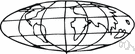# spheroid

(redirected from prolate spheroids)
Also found in: Thesaurus, Medical, Encyclopedia.
Related to prolate spheroids: Prolate ellipsoid

## spher·oid

(sfîr′oid′, sfĕr′-)
n.
A body that is shaped like a sphere but is not perfectly round, especially an ellipsoid that is generated by revolving an ellipse around one of its axes.

sphe′roi·dic′i·ty (-dĭs′ĭ-tē) n.

## spheroid

(ˈsfɪərɔɪd)
n
(Mathematics) maths another name for ellipsoid of revolution
(Mathematics) shaped like but not exactly a sphere

## sphe•roid

(ˈsfɪər ɔɪd)
n.
a solid geometrical figure similar in shape to a sphere, as an ellipsoid.
[1655–65; < Latin sphaeroīdēs < Greek sphairoeidḗs. See sphere, -oid]

## sphe·roid

(sfîr′oid′)
A three-dimensional geometric surface generated by rotating an ellipse on or about one of its axes.
ThesaurusAntonymsRelated WordsSynonymsLegend:
 Noun 1spheroid - a shape that is generated by rotating an ellipse around one of its axes; "it looked like a sphere but on closer examination I saw it was really a spheroid"ellipsoid of revolutionround shape - a shape that is curved and without sharp angles
Translations

[ˈsfɪərɔɪd] N

## spheroid

n (Geometry) → Rotationsellipsoid nt

## sphe·roid

, spheroidal
n. esferoide, de forma esférica.
References in periodicals archive ?
Caption: Figure 1: The prolate spheroidal geometry and the three coordinate surfaces, that is, prolate spheroids ([tau] = const.), hyperboloids of two sheets ([zeta] = const.), and meridian planes ([phi] = const.) with c being the semifocal distance of the coordinate system.
, we have further expanded our studies to Stokes flow problems around nonconvex bodies, such as the inverted prolate spheroids. The obtained expressions were used to model the blood plasma flow past a red blood cell , the sedimentation of a red blood cell , and also the blood plasma flow around two aggregated Low Density Lipoproteins  and the translation of two aggregated Low Density Lipoproteins within blood plasma .
The comparison with the numerical results presented in [4, 5] show that for the sphere with k[rho] = 10 the accuracy of the asymptotic formulas is about 15-20% and for prolate spheroids the accuracy of the asymptotics is much lower.
We will refer to these families of solutions as oblate spheroids and prolate spheroids, respectively, with the understanding that in our case these solutions are not literally axisymmetric ellipsoids of revolution.
3 shows the calculated potential drop between the outlet and inlet of the aperture over the range of the angle between the axis of revolution of a prolate spheroid and the electric field for 4 types of prolate spheroids.
For prolate spheroids ([a.sub.x] > [a.sub.y] = [a.sub.z]) the depolarization factors are,
In the case of spheroids for which two axes are equal (a = b [not equal to] c), the analytical expressions for [A.sub.i] for oblate spheroids (disk-like spheroids with a = b > c) and prolate spheroids (needle-like spheroids with a = b < c) can be found in the literature .

Site: Follow: Share:
Open / Close